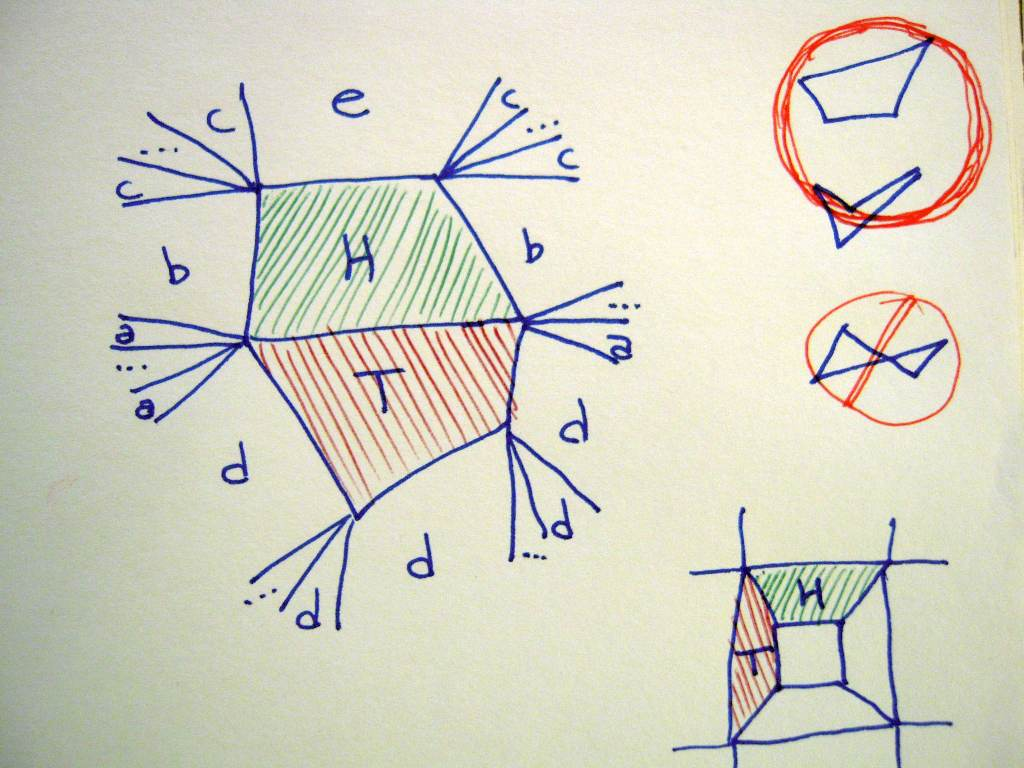mdbtxt1
mdbtxt2
Proceed to Safety

# Gliders on an Infinite Quadrilateral Tiling

Suppose that we have a plane that is completely tiled with quadrilaterals (hereafter called "quads") such that no two quads share more than one edge. The only restriction on their shape is that all the quadrilaterals must be "non-self-crossing", i.e. the edges intersect only at their endpoints (see examples to the right of the figure).

Within this plane, select two quads that share an edge, and call one of them the "head", and the other the "tail". The situation will be as pictured here (H is the head, T is the tail):the head H, tail T, and various types of neighbors

Now consider all of the neighboring quads: those that share either a vertex or an edge with either the head or the tail. These will fall into 5 groups:

• a shares a vertex with both H and T, but does not share an edge with either,
• b shares an edge with H and a vertex with T,
• c shares only a vertex with H, and shares nothing with T,
• e shares only an edge with H, and shares nothing with T,
• d is anything else (any quad that is not a, b, c, e, H or T).

There will be at least three d, there can be any number of a and c, there will be two b, and there will always be exactly one e. Let e be the new H, and let the old H be the new T. Repeat indefinitely.

The above rules can be encoded in a cellular automaton, provided it is able to distinguish whether a given cell shares an edge or just shares a vertex (i.e. you need both the "Moore neighborhood" and the "von Neumann neighborhood"). If you only have one type of neighborhood, it's still possible but you need to use more than 3 states (for example, Adam Goucher did it with 4 states using just the Moore neighborhood). It is not necessary that there be a finite number of different configurations of neighborhoods.

Thus we have an object that moves continuously, but it does not necessarily move indefinitely in any particular direction. An example of a looping path is shown in the lower-right.

Now consider a "Penrose rhomb tiling", such as seen here. In such a tiling, every quad is a parallelogram. After selecting the initial H and T, define perpendicular coordinate axes x and y with the x axis parallel to the shared edge between H and T, and the y axis increasing "in the direction towards H and away from T". Then we know that every time we select a new H, the shared edge between the new H and T will be at a higher y coordinate, and so the pattern cannot ever loop or cross its own path. However, there is no guarantee that it will travel in any particular direction, depending on the grid it could wander anywhere within the half-plane whose y coordinates are higher than the current position of H.

This argument can also apply to many trapezoid tilings, though not all, as the simple looping counterexample above shows. The important requirement is that the new shared edge always be parallel to the previous shared edge.

This page was written in the "embarrassingly readable" markup language RHTF, and was last updated on 2012 Jul 28.s.27